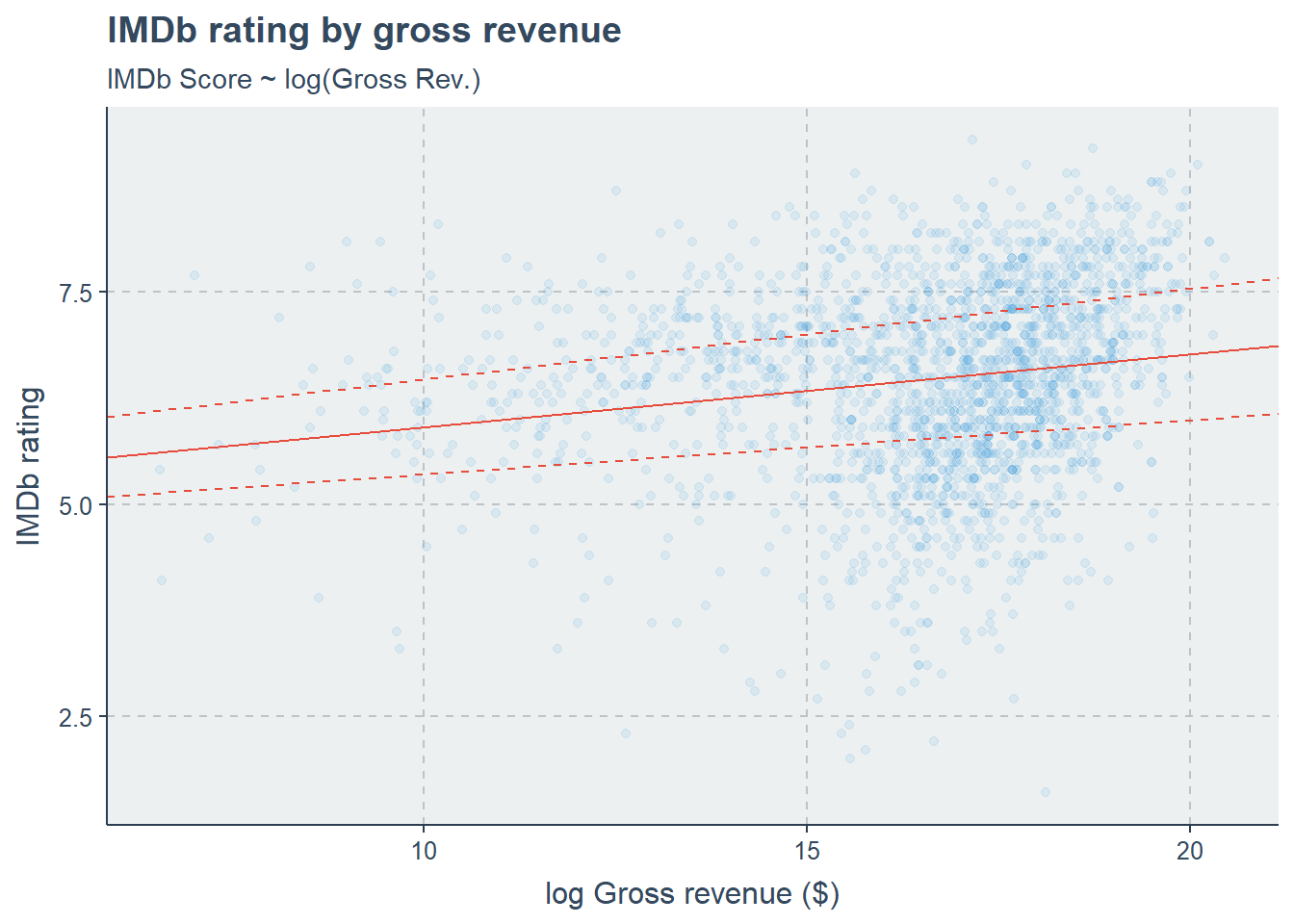## Upcoming Assignments/Quizzes

Assignments Open Time Due Time
Module 11 Data Quiz November 9th (1:00 am EST) November 11th (11:55 pm EST)
Module 11 Conceptual Quiz November 9th (1:00 am EST) November 11th (11:55 pm EST)

## Notes on Simple and Multiple Linear Regression

One of the more common requests/comments we get from students is a desire to see more and different examples. So for this week’s TA notes I’ll go through some of the concepts related to linear regression we’ve learned in the past two modules.

You’ll notice that I may use some different methods for subsetting and maniputlating data and plotting than we’ve covered in the course materials. There is always more than one way to do things in R, but these shouldn’t impact our final results and sometimes it’s helpful to see a different approach.

I’m going to use a couple of packages from the “tidyverse” suite of R packages. I like using these packages because I find the code easier to read and plots prettier than base R.

# Run this line if you have not installed these packages:
# install.packages(c("dplyr", "ggplot2"))

library(dplyr)   # For dataframe manipulation
library(ggplot2) # For nice plots

For this exercise, we’re going to use simple and multiple linear regression to model IMDB scores for movies from their top 5,00 movies (as of 2016). Here’s is the code to load this data into R and format the columns we need:

imdb <- read_csv("https://query.data.world/s/53o5lmqz56eb6pxsdc3qzh5glfavkv") %>%
select(title = movie_title, score = imdb_score, budget, gross, duration,
release_year = title_year, fb_likes = movie_facebook_likes,
rating = content_rating) %>%
na.omit() %>%                           # Removes NAs
filter_if(is.numeric, all_vars(. > 0))  # Removes rows with zeros
title score budget gross duration release_year fb_likes rating
Avatar 7.9 237000000 760505847 178 2009 33000 PG-13
Spectre 6.8 245000000 200074175 148 2015 85000 PG-13
The Dark Knight Rises 8.5 250000000 448130642 164 2012 164000 PG-13
John Carter 6.6 263700000 73058679 132 2012 24000 PG-13
Tangled 7.8 260000000 200807262 100 2010 29000 PG
Avengers: Age of Ultron 7.5 250000000 458991599 141 2015 118000 PG-13

Some of this code may look a little strange. The biggest difference is the %>%, which is called a “pipe”. The pipe is a special operator that comes from the magrittr package (dplyr is calling it for us here), and what it does is take the output from whatever comes before the pipe and puts it into the the first argument of the next function.

This is useful when using the dpylr package, which is set up to string together functions, kind of like verbs in a sentence. This does two things, it means we don’t need to make intermediate objects that won’t be used and it makes the code easier for humans to read. The code above can be “read” as:

First read a CSV from this location, then select these columns, then omit rows with NA, and finally filter out rows with zeros.

Looking at our data, our response variables is going to be imdb$score, and we have a few different predictors: film budget, gross revenue made, duration, year of release, and even Facebook likes! ### Checking correlations First things first, let’s look at the correlations in our data. This will help us get an idea for which predictor variables are highly associated with our response variable, and tell which covariates are correlated (which may be important for our multiple regressions). imdb %>% select(- c(title, rating)) %>% # remove unneeded columns cor() %>% round(2) score budget gross duration release_year fb_likes score 1.00 0.07 0.28 0.40 -0.05 0.38 budget 0.07 1.00 0.41 0.22 0.18 0.25 gross 0.28 0.41 1.00 0.29 0.13 0.47 duration 0.40 0.22 0.29 1.00 -0.08 0.31 release_year -0.05 0.18 0.13 -0.08 1.00 0.36 fb_likes 0.38 0.25 0.47 0.31 0.36 1.00 We can visualize these correlations using the ggcorrplot package: ggcorrplot::ggcorrplot( imdb %>% select(- c(title, rating)) %>% cor(), type = "lower", method = "circle", lab = T )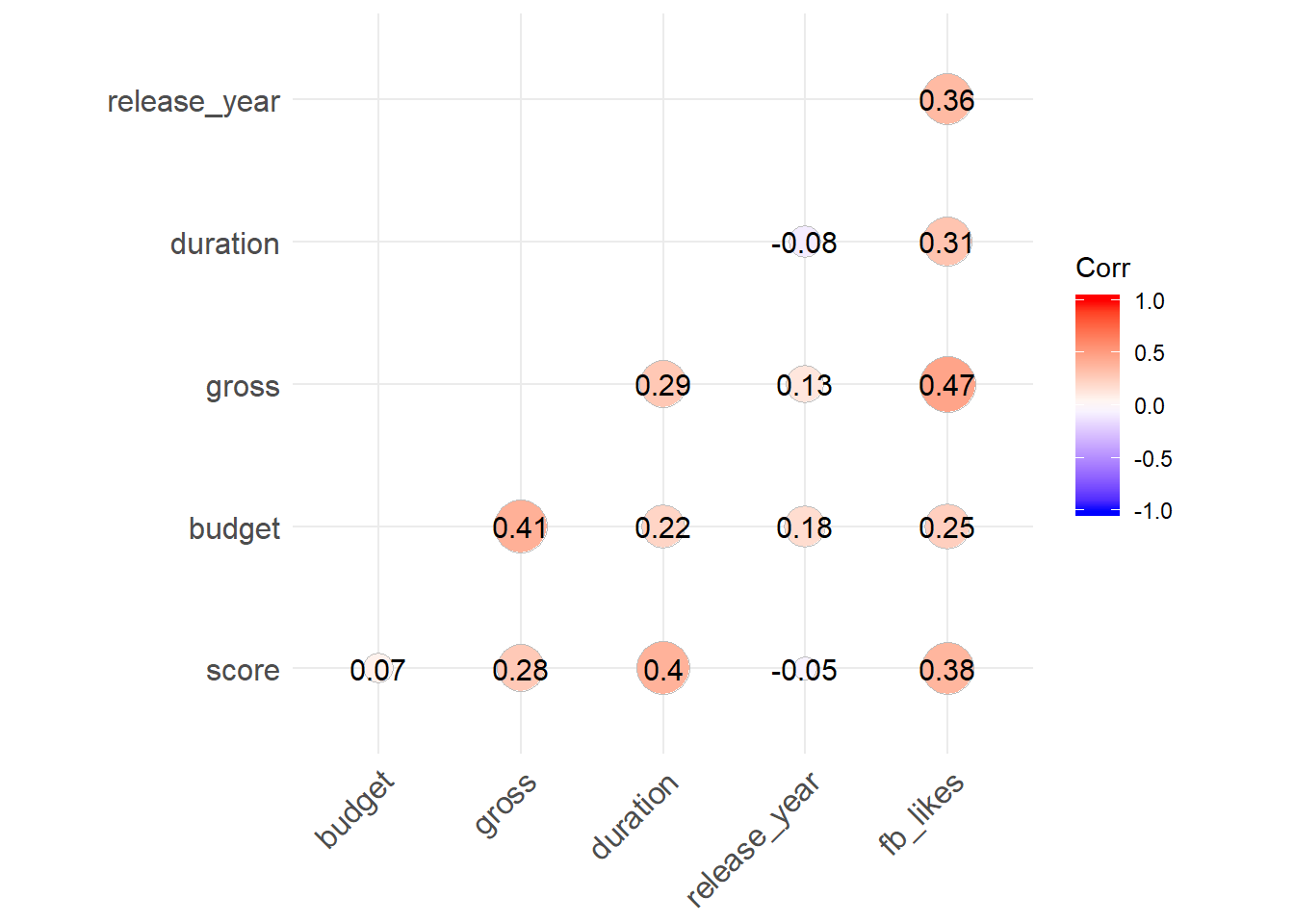### Simple linear regression One hypothesis could be that movies that make more money should have a hinger rating, so let’s use a simple linear regresion using gross revenue imdb$gross to predict IMDB score imdb$score. First, we’ll look at these varibles using a LOESS regression. ggplot(imdb, aes(gross, score)) + geom_point(alpha = 0.2) + geom_smooth(method = "loess", se = FALSE, size = 1.5) + labs(x = "Gross revenue ($)", y = "IMDb rating",
title = "IMDb rating by gross revenue",
subtitle = "LOESS regression")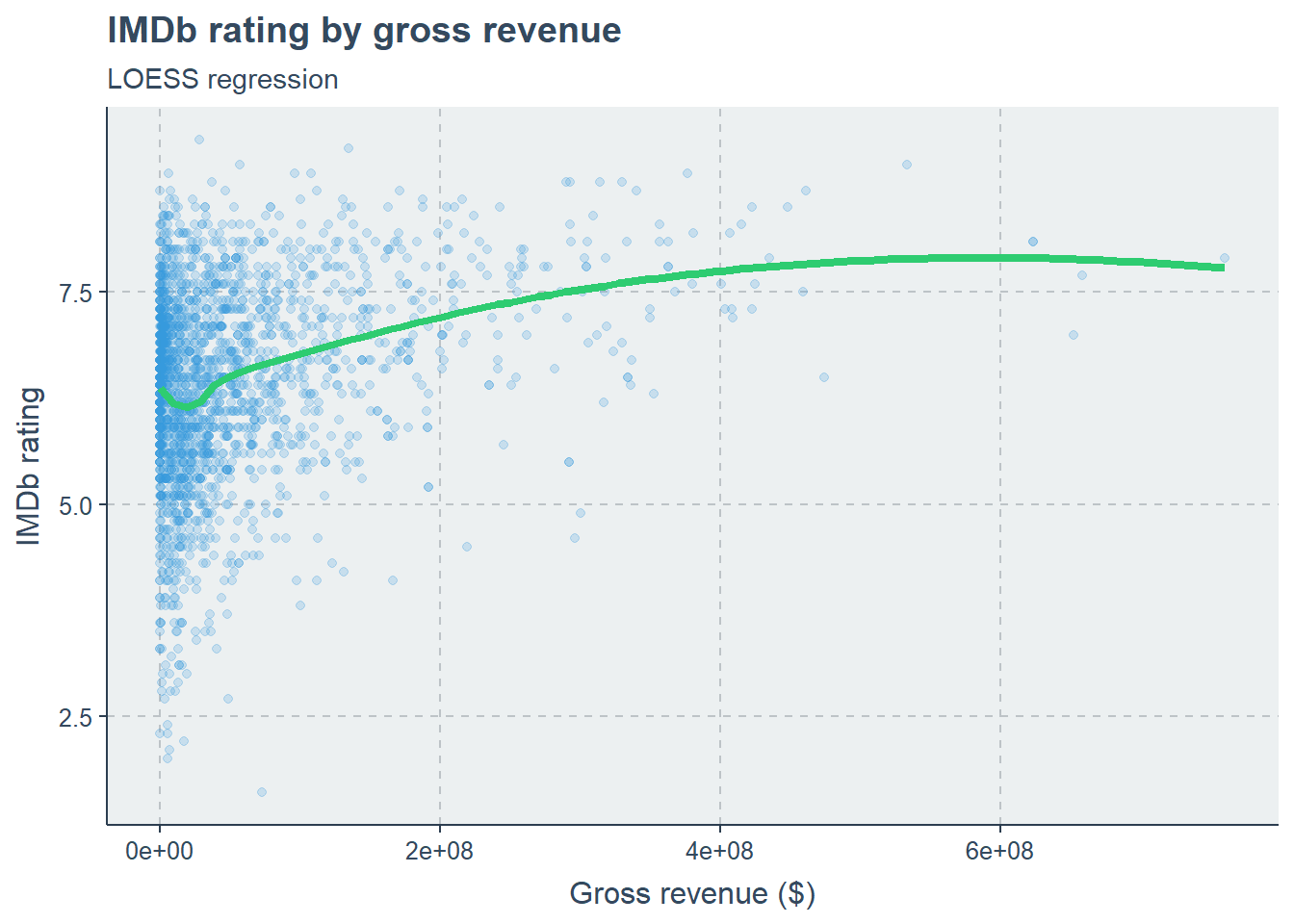Hard to say if this will be a good predictors, there are a lot of low budget films! Let’s make the linear model and check some assumptions:

model <- lm(score ~ gross, data = imdb)

# Check assumptions
# Plot fitted vs. residuals
ggplot() +
geom_point(aes(x = model$fitted.values, y = model$residuals)) +
geom_hline(yintercept = 0, color = "black", linetype = 2) +
labs(title = "Fitted vs. Residuals",
subtitle = "IMDb Score ~ Gross Rev.",
x = "Fitted values",
y = "Residuals")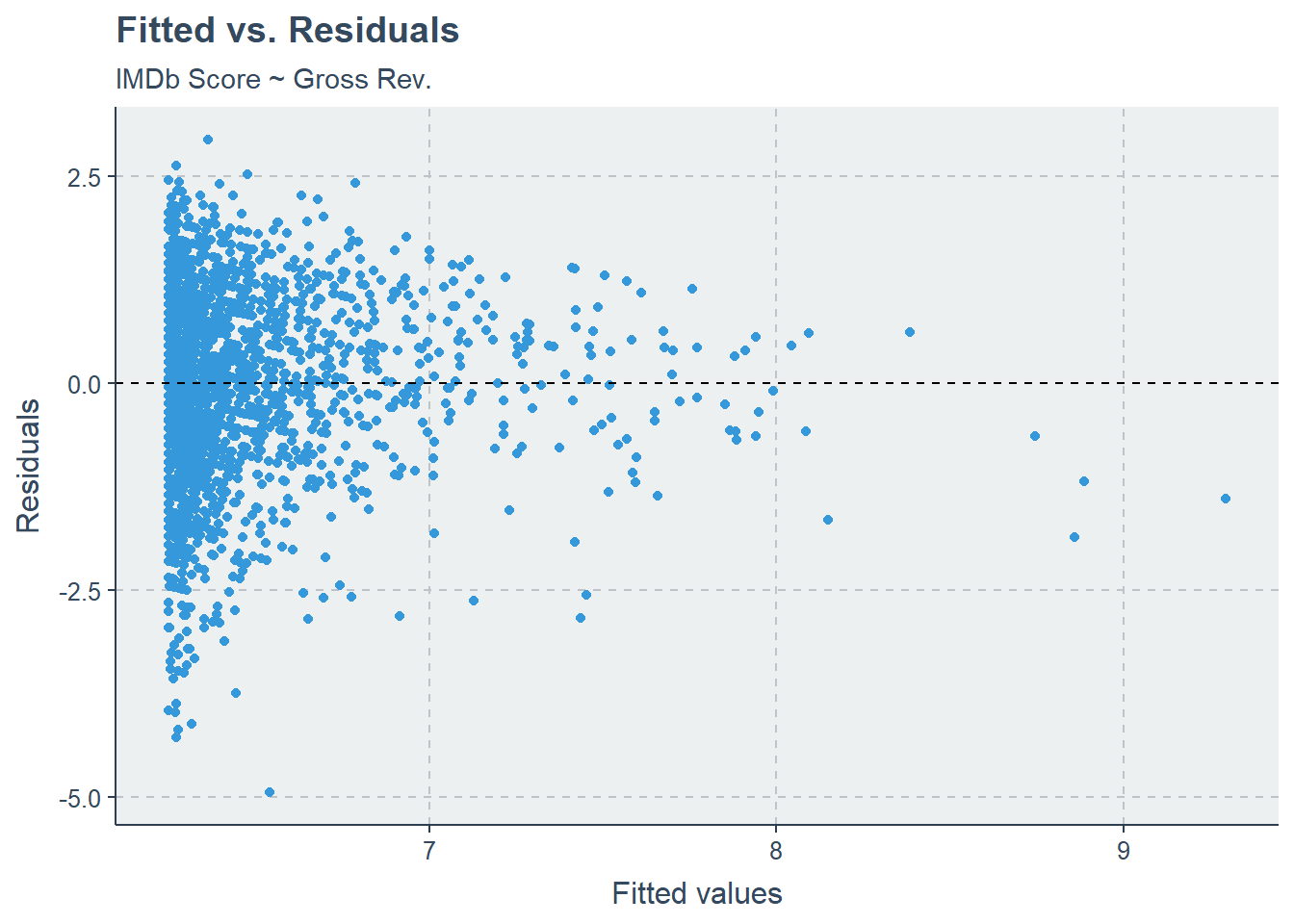# QQ-plot
ggplot() +
stat_qq(aes(sample = rstandard(model))) +
stat_qq_line(aes(sample = rstandard(model))) +
labs(title = "Q-Q plot",
subtitle = "IMDb Score ~ Gross Rev.")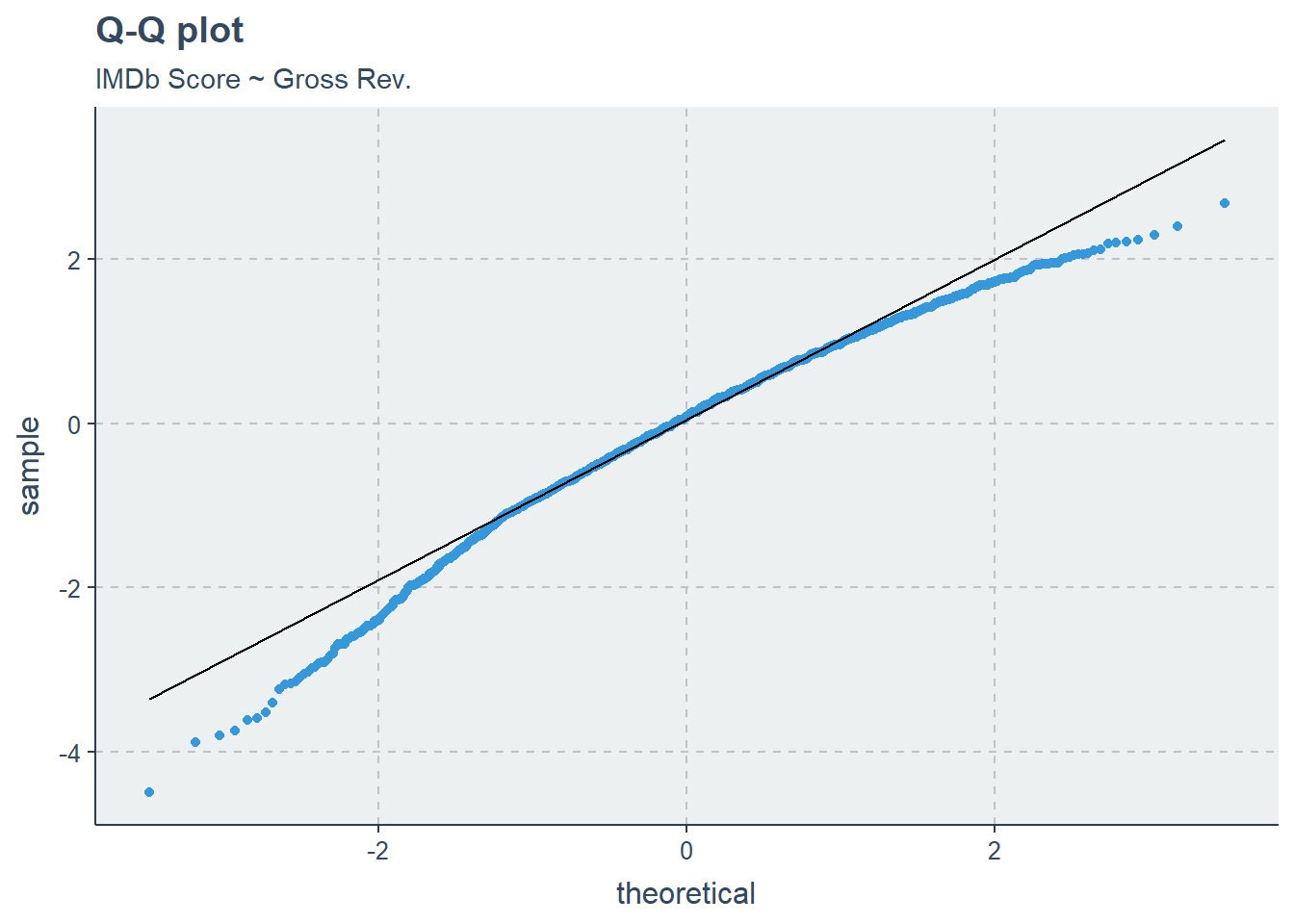Our linear assumptions are not exactly what we would hope, likely due to the skewness of our predictor variable:

ggplot(imdb) +
geom_histogram(aes(gross)) +
labs(title = "Gross revenue is heavily right-skewed",
x = "Gross revenue ($)", y = "") ## stat_bin() using bins = 30. Pick better value with binwidth.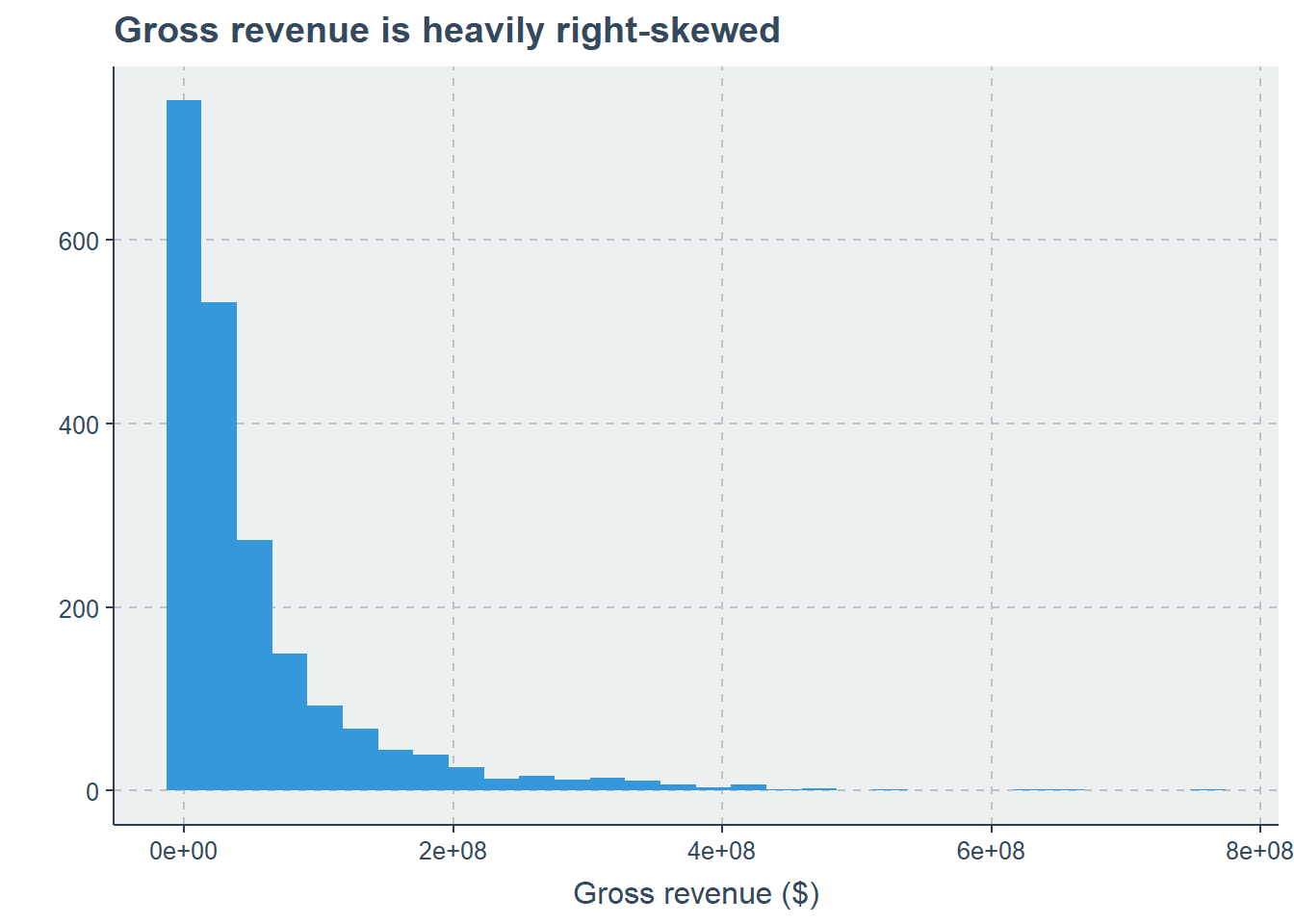We can try a log transformation to see if this better fits our assumptions? # Try the log transform ggplot(imdb, aes(gross, score)) + geom_point(alpha = 0.2) + geom_smooth(method = "loess", se = FALSE, size = 1.5) + scale_x_log10() + labs(x = "Gross revenue ($)", y = "IMDb rating",
title = "IMDb rating by gross revenue",
subtitle = "LOESS regression")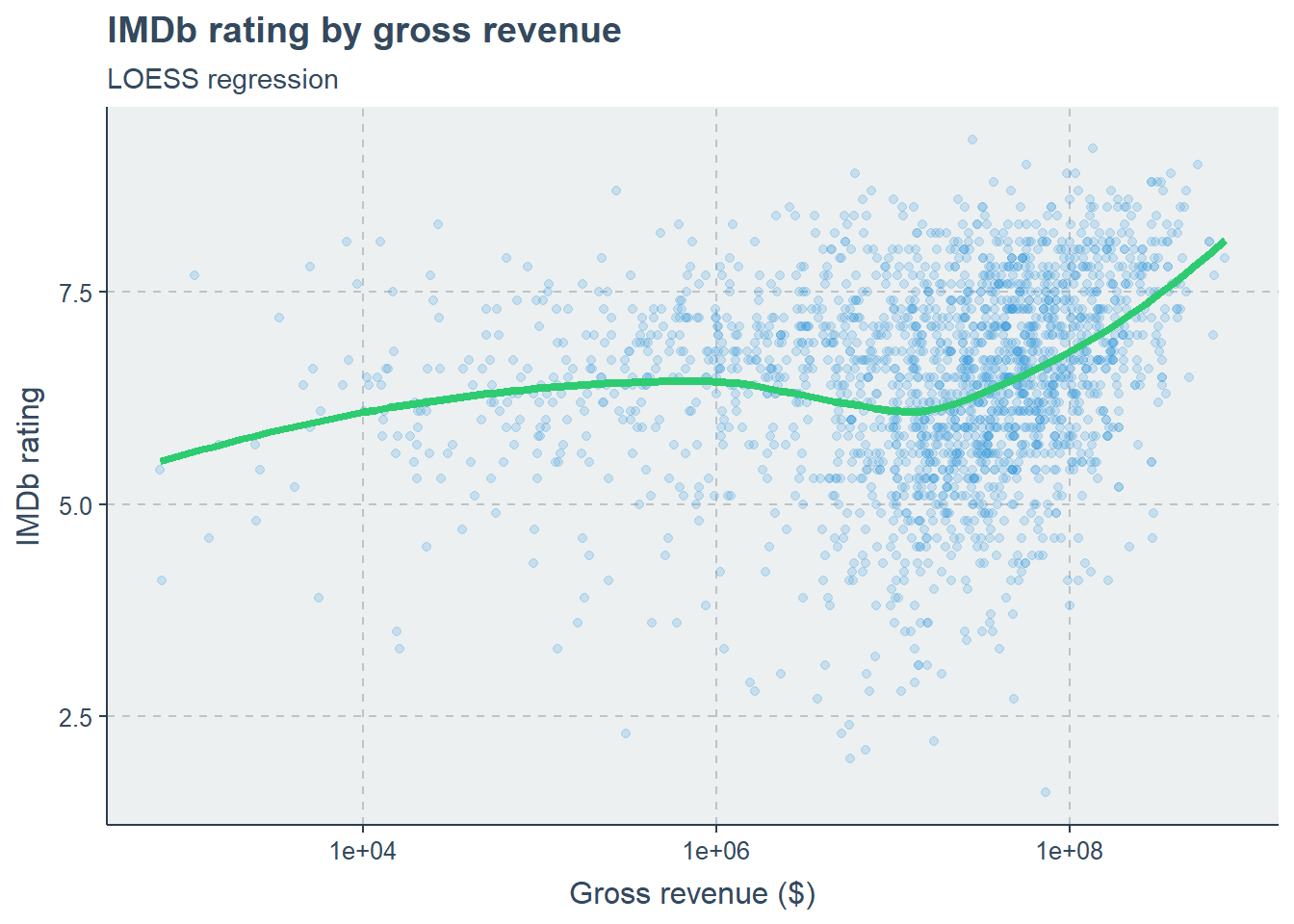ggplot(imdb) +
geom_histogram(aes(log(gross))) +
labs(title = "log of Gross revenue is less skewed",
x = "log(Gross revenue ($))", y = "") ## stat_bin() using bins = 30. Pick better value with binwidth.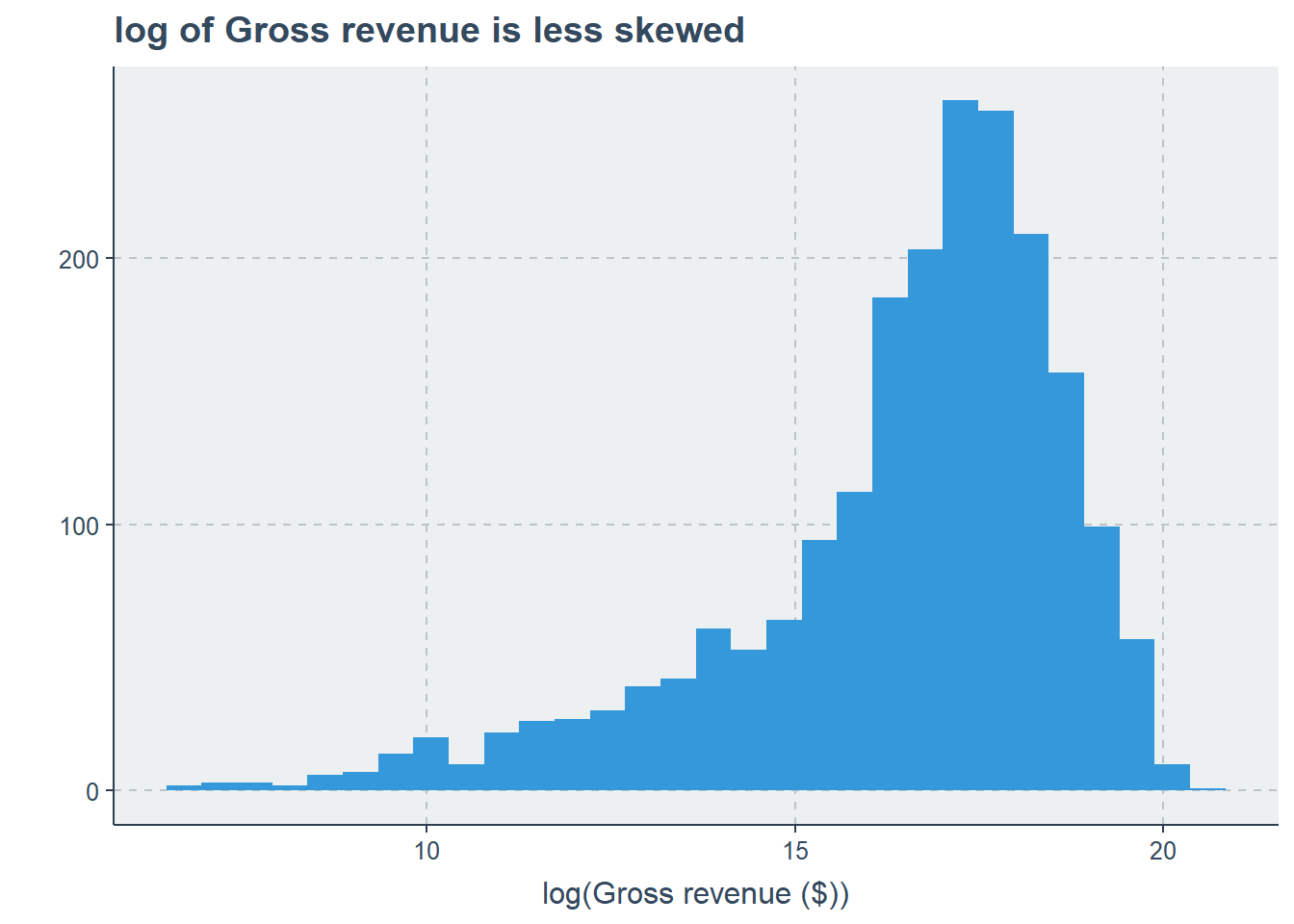# Make new lm log_model <- lm(score ~ log(gross), data = imdb) # Check assumptions # Plot fitted vs. residuals ggplot() + geom_point(aes(x = log_model$fitted.values, y = log_model$residuals)) + geom_hline(yintercept = 0, color = "black", linetype = 2) + labs(title = "Fitted vs. Residuals", subtitle = "IMDb Score ~ log(Gross Rev.)", x = "Fitted values", y = "Residuals")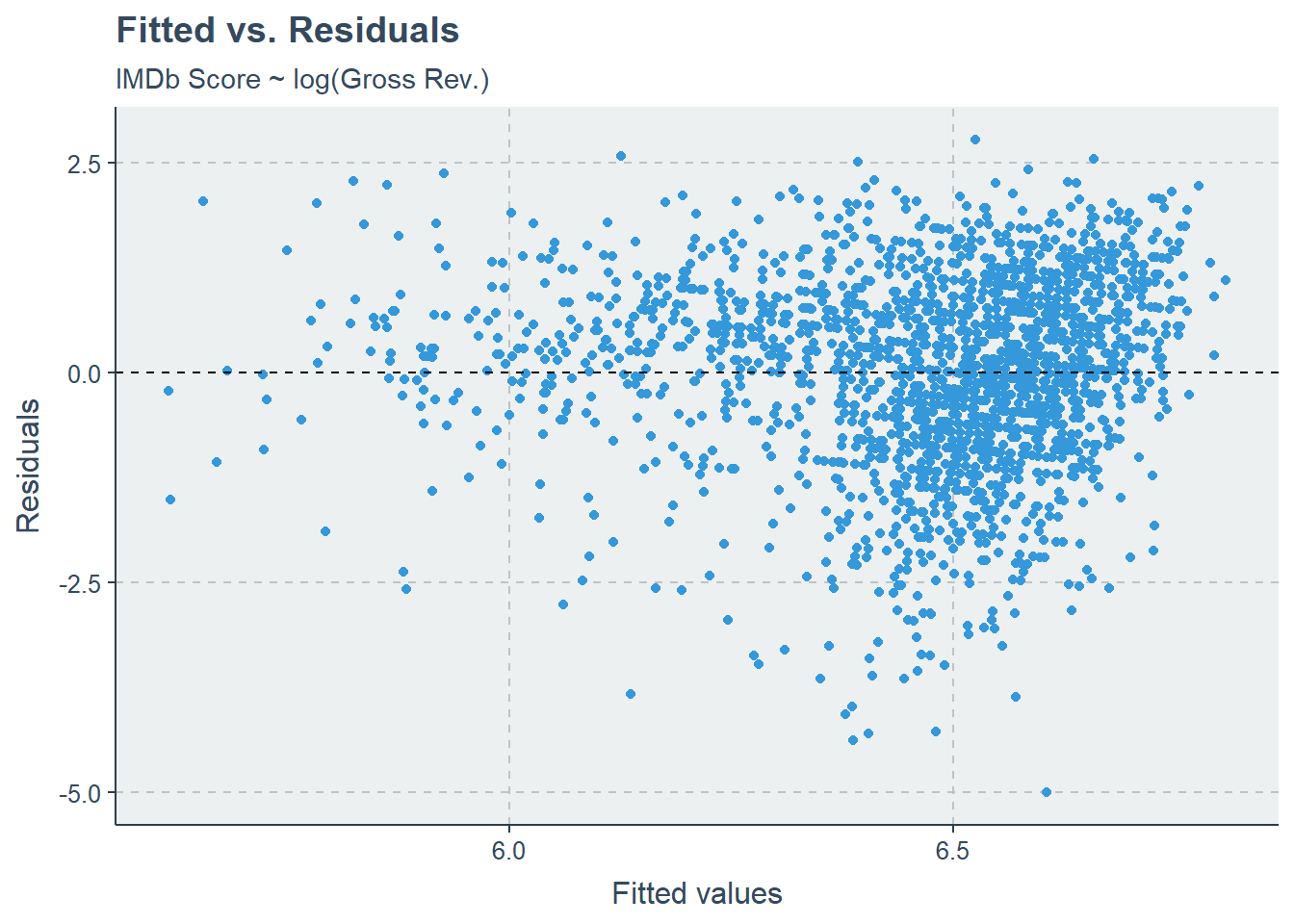# QQ-plot ggplot() + stat_qq(aes(sample = rstandard(log_model))) + stat_qq_line(aes(sample = rstandard(log_model))) + labs(title = "Q-Q plot", subtitle = "IMDb Score ~ log(Gross Rev.)")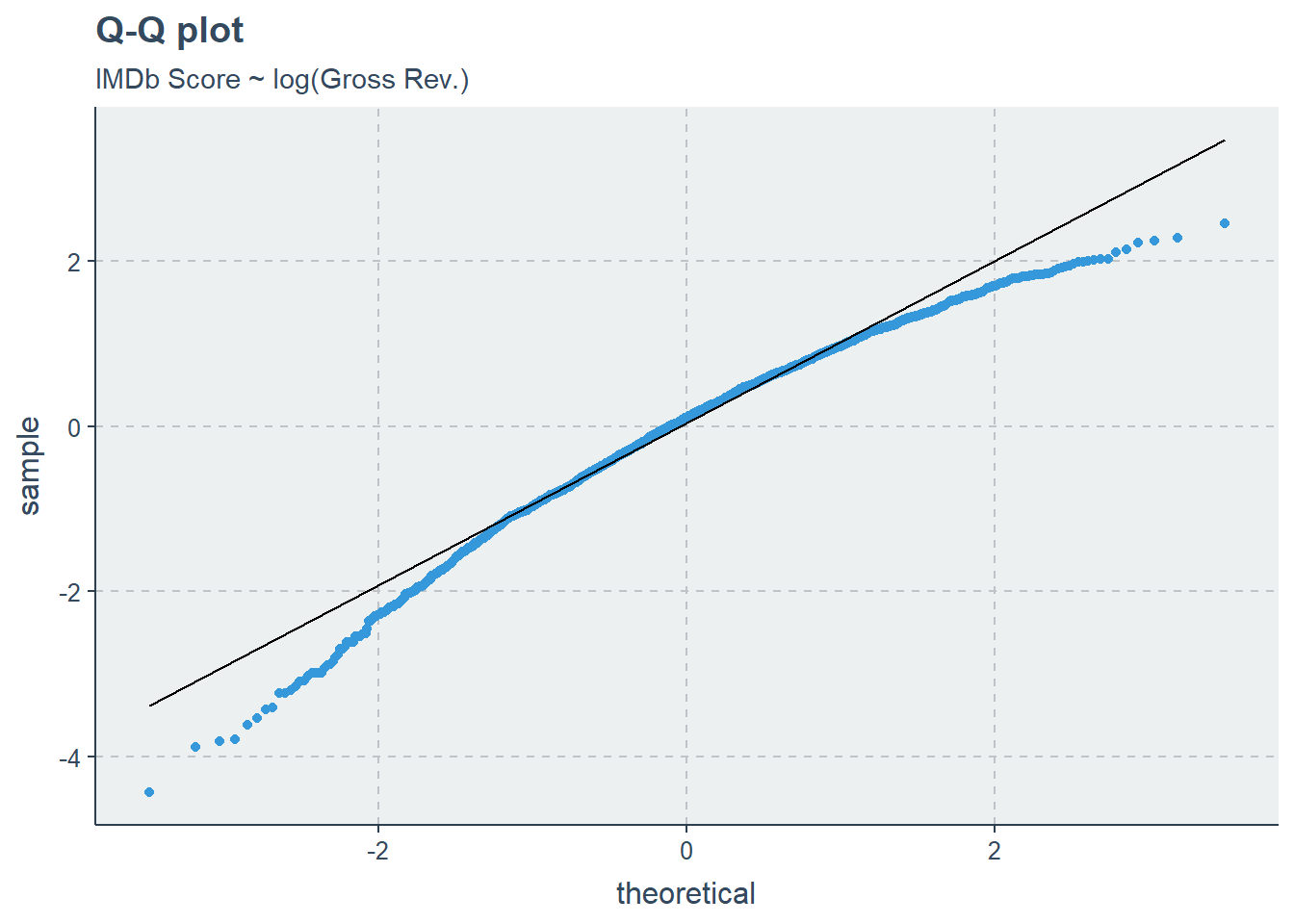This looks a little better, now let’s interpret some of these results. anova(log_model) ## Analysis of Variance Table ## ## Response: score ## Df Sum Sq Mean Sq F value Pr(>F) ## log(gross) 1 80.65 80.645 63.351 2.824e-15 *** ## Residuals 2070 2635.10 1.273 ## --- ## Signif. codes: 0 '***' 0.001 '**' 0.01 '*' 0.05 '.' 0.1 ' ' 1 summary(log_model) ## ## Call: ## lm(formula = score ~ log(gross), data = imdb) ## ## Residuals: ## Min 1Q Median 3Q Max ## -5.0053 -0.6923 0.1203 0.8018 2.7758 ## ## Coefficients: ## Estimate Std. Error t value Pr(>|t|) ## (Intercept) 5.05447 0.17880 28.269 < 2e-16 *** ## log(gross) 0.08565 0.01076 7.959 2.82e-15 *** ## --- ## Signif. codes: 0 '***' 0.001 '**' 0.01 '*' 0.05 '.' 0.1 ' ' 1 ## ## Residual standard error: 1.128 on 2070 degrees of freedom ## Multiple R-squared: 0.0297, Adjusted R-squared: 0.02923 ## F-statistic: 63.35 on 1 and 2070 DF, p-value: 2.824e-15 These tables tell us all sorts of useful information (sum of squares, mean square error, etc.). Perhaps most importantly, we see that the slope estimate has an assocaited p-value < 0.05, and that it is positive. This suggests that movies that make more money do have higher IMDb ratings! However, it is important to note that we’ve log-transformed our predictor variables, which makes it less clear to interpet. Now let’s plot these findings. m <- summary(log_model) ggplot(imdb) + geom_point(aes(log(gross), score), alpha = 0.1) + geom_abline(intercept = m$coefficients[1,1],
slope = m$coefficients[2,1], color = "#e74c3c") + # Let's also add the 95% confidence interval geom_abline(intercept = m$coefficients[1,1] - 1.96*m$coefficients[1,2], slope = m$coefficients[2,1] - 1.96*m$coefficients[2,2], color = "#e74c3c", linetype = 2) + geom_abline(intercept = m$coefficients[1,1] + 1.96*m$coefficients[1,2], slope = m$coefficients[2,1] + 1.96*m$coefficients[2,2], color = "#e74c3c", linetype = 2) + labs(x = "log Gross revenue ($)", y = "IMDb rating",
title = "IMDb rating by gross revenue",
subtitle = "IMDb Score ~ log(Gross Rev.)")